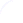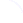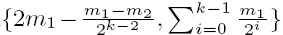Search
 Submission Procedure share: |Follow usavailable in:   PDF (122 kB) PS (153 kB)

get:
 Similar Docs BibTeX Write a comment
get:
 Links into Future

### A Linear Time Approximation Algorithm for Ruler Folding Problem

Ali Nourollah (Amirkabir University of Technology, Iran)

Mohammadreza Razzazi (Amirkabir University of Technology, Iran)

Abstract: A chain or n-link is a sequence of n links whose lengths are fixed and are joined together from their endpoints, free to turn about their endpoints, which act as joints. "Ruler Folding Problem", which is NP-Complete is to find the minimum length of the folded chain. The best linear approximation algorithm for it were proposed by Hopcroft et al. Their algorithm folds any open chain in the interval whose length is less than 2m1, where m1 is the length of the longest link in the chain. We propose a linear time approximation algorithm using O(1) additional space. Our algorithm has lower upper bound for the length of the folded chain which is max, where m1 and m2are the lengths of the two distinct maximum length links in the chain respectively, and k is the number of links whose lengths are m1 in the chain. Hence it is the best known approximation algorithm for "Ruler Folding Problem".

Keywords: Carpenter's Ruler, Ruler Folding Problem, approximation algorithms

Categories: F.2, G.2.1